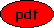Singular Initial Value Problems for Quasi-Linear Ordinary Differential Equations Co-author(s): Matthias Seiß Reference: Journal of Differential Equations, 281 (2021) 258-288 Description: We study initial value problems of scalar quasi-linear ordinary differential equations where the initial point is an impasse point of the equation. We first recall some notions from the classical geometric singularity theory of differential equations and then show that quasi-linear equations need their own theory leading to the notion of an impasse point. In particular, we demonstrate that there might be impasse points even if no singularities in the classical sense are present. We reduce questions of existence, (non-)uniqueness and regularity of solutions of initial value problems at impasse points to the problem of analysing the locals solution of a vector field around a stationary point, i.e. to a classical problem in dynamical system theory. In the second part of the paper, we use the developed theory for a detailed analysis of the initial value problem of the second-order equation g(x)u''=f(x,u,u') with the initial conditions prescribed at a simple zero of g and recover geometrically the results obtained by Liang with classical analytical techniques. PDF File:(806 kB) Home, Last update: Thu May 27 21:26:48 2021